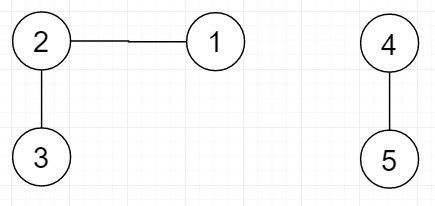# Find all reachable nodes from every node present in a given set in C++

Suppose we have one undirected graph and a set of vertices; we have to find all reachable nodes from every vertex present in the given set.

So, if the input is likethen the output will be [1,2,3] and [4,5] as these are two connected components.

To solve this, we will follow these steps −

• nodes := number of nodes in the graph
• Define an array visited of size: nodes+1. And fill with 0
• Define one map m
• comp_sum := 0
• for initialize i := 0, when i < n, update (increase i by 1), do −
• u := arr[i]
• if visited[u] is false, then −
• (increase comp_sum by 1)
• m[visited[u]] := bfs traversal of g from node u, also calculate comp_sum
• print traversal of m[visited[u]]

## Example

Let us see the following implementation to get better understanding −

Live Demo

#include <bits/stdc++.h>
using namespace std;
class Graph{
public:
int nodes;
Graph(int);
void insert_edge(int, int);
vector<int> BFS(int, int, int []);
};
Graph::Graph(int nodes) {
this->nodes = nodes;
}
void Graph::insert_edge(int u, int v) {
}
vector<int> Graph::BFS(int comp_sum, int src,int visited[]){
queue<int> queue;
queue.push(src);
visited[src] = comp_sum;
vector<int> reachableNodes;
while(!queue.empty()) {
int u = queue.front();
queue.pop();
reachableNodes.push_back(u);
if (!visited[*itr]) {
visited[*itr] = comp_sum;
queue.push(*itr);
}
}
}
return reachableNodes;
}
void displayReachableNodes(int n, unordered_map <int, vector<int> > m) {
vector<int> temp = m[n];
for (int i=0; i<temp.size(); i++)
cout << temp[i] << " ";
cout << endl;
}
void get_all_reachable(Graph g, int arr[], int n) {
int nodes = g.nodes;
int visited[nodes+1];
memset(visited, 0, sizeof(visited));
unordered_map <int, vector<int> > m;
int comp_sum = 0;
for (int i = 0 ; i < n ; i++) {
int u = arr[i];
if (!visited[u]) {
comp_sum++;
m[visited[u]] = g.BFS(comp_sum, u, visited);
}
cout << "Reachable Nodes from " << u <<" are\n";
displayReachableNodes(visited[u], m);
}
}
int main() {
int nodes = 5;
Graph g(nodes);
g.insert_edge(1, 2);
g.insert_edge(2, 3);
g.insert_edge(4, 5);
int arr[] = {2, 4, 1};
int n = sizeof(arr)/sizeof(int);
get_all_reachable(g, arr, n);
}

## Input

g.insert_edge(1, 2);
g.insert_edge(2, 3);
g.insert_edge(4, 5);

## Output

Reachable Nodes from 2 are
2 1 3
Reachable Nodes from 4 are
4 5
Reachable Nodes from 1 are
2 1 3